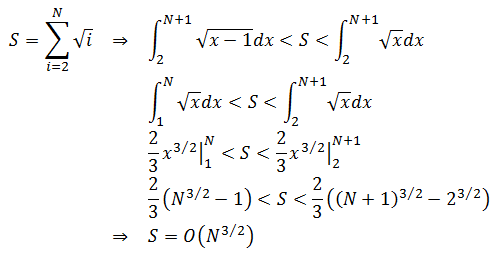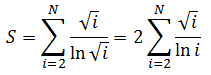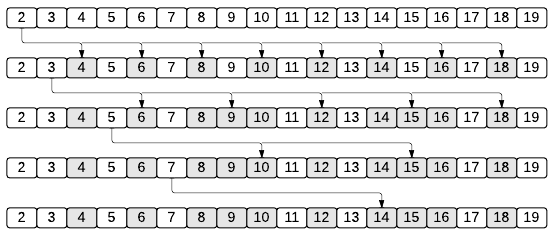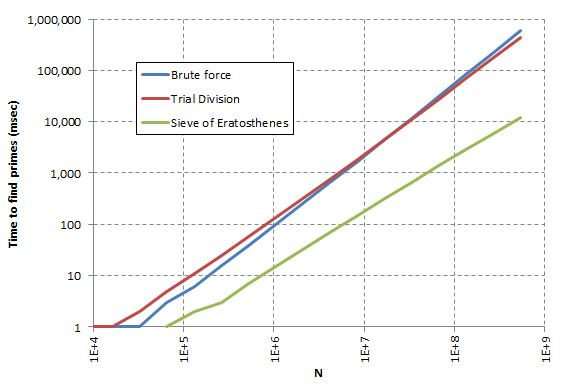# Finding All Prime Numbers Smaller Than Specified Value

by Zoran Horvat

## Problem Statement

Given a value N, N > 2, write a function which returns an array with all prime numbers that are not greater than N.

Examples: For N=20, the function should return array [2, 3, 5, 7, 11, 13, 17, 19]. For N=100, resulting array should be [2, 3, 5, 7, 11, 13, 17, 19, 23, 29, 31, 37, 41, 43, 47, 53, 59, 61, 67, 71, 73, 79, 83, 89, 97].

## Problem Analysis

In the following sections we are going to try several approaches to solving the problem.

### Brute Force Approach

In exercise Testing if Number is Prime , we have argued that it is possible to test whether one number is prime by trying all numbers greater than one which do not exceed its square root as possible divisors. If no divisors are found, then the number is officially declared prime. Thus it seems feasible to simply iterate through all the numbers and test each of them. Then testing each number k takes square root of k to complete. This means that total number of steps required to extract all prime numbers not exceeding N is:Unfortunately, this sum doesn’t have a closed form. But we can still calculate its asymptotic bounds with some help of integrals. Basically, exact value of the sum lies between values of integrals of two functions. First function would be the square root curve which passes under each element of the sum. Second function would again be the square root curve, but the one passing over each element of the sum. To cut the long story short, here is the solution:The function which operates on this principle might look like this:
``````function ExtractPrimesBruteForce(n)
n – largest number to test
begin

primes – empty array

for k = 2 to n
if IsPrime(k) then
append k to primes

return primes

end

function IsPrime(n)
begin
result = false
if n <= 3 then
result = true
else if n mod 2 <> 0 AND n mod 3 <> 0 then
begin

k = 5
step = 2

while k * k <= n AND n mod k <> 0
begin
k = k + step
step = 6 - step
end

if n = k OR n mod k <> 0 then
result = true

end

return result

end
``````

The IsPrime function is copied from Testing if Number is Prime .

### Trial Division Approach

What we could do next is to try to optimize this algorithm. For example, why would we divide one number with all smaller numbers? Since we are finding all prime numbers, we already know the list of all prime numbers smaller than current number. This list is much shorter than the list of all odd numbers not divisible by 3, as the IsPrime function above does. Why don’t we run a test like this:

``````isPrime = true
for each prime in primesCollection
if number mod prime = 0 then
isPrime = false
``````

Let’s see how efficient this loop is. Number of prime numbers smaller than specified value k is well approximated by the following function:Generally, in order to find whether one number is prime, we should try dividing it with prime numbers that do not exceed its square root. Total number of divisions required to extract prime numbers that do not exceed N is then:This sum once again does not have the closed form. Attempting the integration approach as above would apparently lead us to the field of exponential integral functions. But anyway, it is easy to show that this algorithm has sufficient room for performance improvement compared to its predecessor, the brute force algorithm:Conclusion is that improved algorithm, often referred to as trial division, is on a good track of performing better than the brute force algorithm. Below is the pseudocode which implements it.

``````function ExtractPrimesTrial(n)
begin

primes – empty array

for k = 2 to n
begin

isPrime = true

for each m in primes
begin
if m * m > k then
exit loop
else if k mod m = 0 then
isPrime = false
exit loop
end

if isPrime then
append k to primes

end

return primes

end
``````

### Optimized Sieve Algorithm

Next thing that we could do is to reverse the process. In trial division attempt, we were dividing each of the numbers with known primes. What about doing it the other way around? Suppose that we have established one prime number as the fact (e.g. value 2 is a trivial prime). Then we can rule out all its multiples from the list. After that, we could pick up the next remaining number (which would be number 3), conclude that it is prime simply because it was not removed in previous step, and then remove all its successors. Since value 4 has been ruled out by prime number 2, next candidate to expand would be 5. By continuing this process we will remove all multiples of all prime numbers. Numbers that remain will be the prime numbers that we wanted to find.

For example, take a look at the following picture, which demonstrates how we can find all prime numbers smaller than 20.This process promotes numbers 2, 3, 5, 7, 11, 13, 17 and 19 as prime numbers smaller than 20 - which is right. What is not so pleasing about it is that we are apparently working too much. For example, we can easily conclude that there is no point in expanding prime numbers greater than square root of N. Any value obtained would already have been scratched by some smaller prime number.

Second improvement is more subtle. Suppose that we are about to expand some number k by scratching numbers 2k, 3k, 4k, … until N is breached. Now consider multiples mk such that m < k. These multiples have already been scratched by number m (if m is prime) or by smallest of its factors (if m is composite). So, expanding any prime number k would effectively start from k^2 and then move on to k^2+k, k^2+2k, etc.

This algorithm is known as the Sieve of Eratosthenes, after ancient Greek mathematician Eratosthenes of Cyrene. Asymptotic complexity of the algorithm is O(N loglogN). And below is the pseudocode.

``````function SieveOfEratosthenes(n)
begin

isPrime – array of flags
for i = 2 to n
isPrime[i] = true

primes – array of primes

for k = 2 to N
if isPrime[k] then
begin
append k to primes
m = k * k
while m <= n
begin
isPrime[m] = false
m = m + k
end
end

return primes

end
``````

## Implementation

Below is the source code of console application in C# which implements all three algorithms for finding prime numbers.

``````using System;
using System.Collections.Generic;

{

public class Program
{

delegate int[] ExtractPrimesDelegate(int n);

static int[] ExtractPrimesBruteForce(int n)
{

List<int> primes = new List<int>();

for (int k = 2; k <= n; k++)
if (IsPrime(k))

return primes.ToArray();

}

static bool IsPrime(int n)
{

bool isPrime = false;

if (n <= 3)
{
isPrime = true;
}
else if (n % 2 != 0 && n % 3 != 0)
{

int k = 5;
int step = 2;

while (k * k <= n && n % k != 0)
{
k += step;
step = 6 - step;
}

if (n == k || n % k != 0)
isPrime = true;

}

return isPrime;

}

static int[] ExtractPrimesTrial(int n)
{

List<int> primes = new List<int>();

for (int k = 2; k <= n; k++)
{

bool isPrime = true;

foreach (int prime in primes)
{
if (prime * prime > k)
{
break;
}
else if (k % prime == 0)
{
isPrime = false;
break;
}
}

if (isPrime)

}

return primes.ToArray();

}

static int[] ExtractPrimesEratosthenes(int n)
{

bool[] isPrime = new bool[n + 1];
for (int i = 2; i <= n; i++)
isPrime[i] = true;

List<int> primes = new List<int>();

int maxK = (int)Math.Truncate(Math.Sqrt(n));

for (int k = 2; k <= n; k++)
{
if (isPrime[k])
{

if (k <= maxK)
{

int m = k * k;

while (m <= n)
{
isPrime[m] = false;
m += k;
}

}

}
}

return primes.ToArray();

}

static void PrintArray(int[] primes)
{

for (int i = 0; i < primes.Length; i++)
{
if (i > 0 && i % 10 == 0)
Console.WriteLine();
Console.Write("{0,5}", primes[i]);
}

Console.WriteLine();

}

static void RunPerformanceTestsIfRequired()
{

Console.Write("Run performance test? (Y/N) ");
bool runTest = (runTestAnswer.ToUpper() == "Y");

if (runTest)
RunPerformanceTests();

}

static void RunPerformanceTests()
{

ExtractPrimesDelegate[] delegates = new ExtractPrimesDelegate[]
{
ExtractPrimesBruteForce,
ExtractPrimesTrial,
ExtractPrimesEratosthenes
};

Console.WriteLine("N\tBrute force\tTrial Division\tSieve of Eratosthenes");

for (int power = 1; power <= 29; power++)
RunPerformanceTest(delegates, power);

}

static void RunPerformanceTest(ExtractPrimesDelegate[] delegates, int power)
{

int n = 1 << power;
Console.Write(n);

for (int alg = 0; alg < delegates.Length; alg++)
RunPerformanceTestOnAlgorithm(delegates[alg], n);

Console.WriteLine();

}

static void RunPerformanceTestOnAlgorithm(ExtractPrimesDelegate algorithm, int n)
{
int msec = MsecToExecute(algorithm, n);
Console.Write("\t{0}", msec);
}

static int MsecToExecute(ExtractPrimesDelegate dlg, int n)
{

int totalSteps = 10000000;
int rounds = (totalSteps + n - 1)  n;

DateTime tsStart = DateTime.Now;

for (int round = 0; round < rounds; round++)
{
dlg(n);
}

DateTime tsEnd = DateTime.Now;
TimeSpan tspan = tsEnd - tsStart;
double msecPerTest = tspan.TotalMilliseconds  rounds;
int msec = (int)Math.Round(msecPerTest);

return msec;

}

static void Main(string[] args)
{

RunPerformanceTestsIfRequired();

while (true)
{

Console.WriteLine();
Console.Write("Enter N (zero to exit): ");
int n = int.Parse(Console.ReadLine());

if (n <= 0)
break;

int[] primes = ExtractPrimesEratosthenes(n);

PrintArray(primes);

}

}

}
}
``````

## Demonstration

When application above is run, it produces the following output:

```            ```
Run performance test? (Y/N) N

Enter N (zero to exit): 20
2    3    5    7   11   13   17   19

Enter N (zero to exit): 1000
2    3    5    7   11   13   17   19   23   29
31   37   41   43   47   53   59   61   67   71
73   79   83   89   97  101  103  107  109  113
127  131  137  139  149  151  157  163  167  173
179  181  191  193  197  199  211  223  227  229
233  239  241  251  257  263  269  271  277  281
283  293  307  311  313  317  331  337  347  349
353  359  367  373  379  383  389  397  401  409
419  421  431  433  439  443  449  457  461  463
467  479  487  491  499  503  509  521  523  541
547  557  563  569  571  577  587  593  599  601
607  613  617  619  631  641  643  647  653  659
661  673  677  683  691  701  709  719  727  733
739  743  751  757  761  769  773  787  797  809
811  821  823  827  829  839  853  857  859  863
877  881  883  887  907  911  919  929  937  941
947  953  967  971  977  983  991  997

Enter N (zero to exit): 5000
2    3    5    7   11   13   17   19   23   29
31   37   41   43   47   53   59   61   67   71
73   79   83   89   97  101  103  107  109  113
127  131  137  139  149  151  157  163  167  173
179  181  191  193  197  199  211  223  227  229
233  239  241  251  257  263  269  271  277  281
283  293  307  311  313  317  331  337  347  349
353  359  367  373  379  383  389  397  401  409
419  421  431  433  439  443  449  457  461  463
467  479  487  491  499  503  509  521  523  541
547  557  563  569  571  577  587  593  599  601
607  613  617  619  631  641  643  647  653  659
661  673  677  683  691  701  709  719  727  733
739  743  751  757  761  769  773  787  797  809
811  821  823  827  829  839  853  857  859  863
877  881  883  887  907  911  919  929  937  941
947  953  967  971  977  983  991  997 1009 1013
1019 1021 1031 1033 1039 1049 1051 1061 1063 1069
1087 1091 1093 1097 1103 1109 1117 1123 1129 1151
1153 1163 1171 1181 1187 1193 1201 1213 1217 1223
1229 1231 1237 1249 1259 1277 1279 1283 1289 1291
1297 1301 1303 1307 1319 1321 1327 1361 1367 1373
1381 1399 1409 1423 1427 1429 1433 1439 1447 1451
1453 1459 1471 1481 1483 1487 1489 1493 1499 1511
1523 1531 1543 1549 1553 1559 1567 1571 1579 1583
1597 1601 1607 1609 1613 1619 1621 1627 1637 1657
1663 1667 1669 1693 1697 1699 1709 1721 1723 1733
1741 1747 1753 1759 1777 1783 1787 1789 1801 1811
1823 1831 1847 1861 1867 1871 1873 1877 1879 1889
1901 1907 1913 1931 1933 1949 1951 1973 1979 1987
1993 1997 1999 2003 2011 2017 2027 2029 2039 2053
2063 2069 2081 2083 2087 2089 2099 2111 2113 2129
2131 2137 2141 2143 2153 2161 2179 2203 2207 2213
2221 2237 2239 2243 2251 2267 2269 2273 2281 2287
2293 2297 2309 2311 2333 2339 2341 2347 2351 2357
2371 2377 2381 2383 2389 2393 2399 2411 2417 2423
2437 2441 2447 2459 2467 2473 2477 2503 2521 2531
2539 2543 2549 2551 2557 2579 2591 2593 2609 2617
2621 2633 2647 2657 2659 2663 2671 2677 2683 2687
2689 2693 2699 2707 2711 2713 2719 2729 2731 2741
2749 2753 2767 2777 2789 2791 2797 2801 2803 2819
2833 2837 2843 2851 2857 2861 2879 2887 2897 2903
2909 2917 2927 2939 2953 2957 2963 2969 2971 2999
3001 3011 3019 3023 3037 3041 3049 3061 3067 3079
3083 3089 3109 3119 3121 3137 3163 3167 3169 3181
3187 3191 3203 3209 3217 3221 3229 3251 3253 3257
3259 3271 3299 3301 3307 3313 3319 3323 3329 3331
3343 3347 3359 3361 3371 3373 3389 3391 3407 3413
3433 3449 3457 3461 3463 3467 3469 3491 3499 3511
3517 3527 3529 3533 3539 3541 3547 3557 3559 3571
3581 3583 3593 3607 3613 3617 3623 3631 3637 3643
3659 3671 3673 3677 3691 3697 3701 3709 3719 3727
3733 3739 3761 3767 3769 3779 3793 3797 3803 3821
3823 3833 3847 3851 3853 3863 3877 3881 3889 3907
3911 3917 3919 3923 3929 3931 3943 3947 3967 3989
4001 4003 4007 4013 4019 4021 4027 4049 4051 4057
4073 4079 4091 4093 4099 4111 4127 4129 4133 4139
4153 4157 4159 4177 4201 4211 4217 4219 4229 4231
4241 4243 4253 4259 4261 4271 4273 4283 4289 4297
4327 4337 4339 4349 4357 4363 4373 4391 4397 4409
4421 4423 4441 4447 4451 4457 4463 4481 4483 4493
4507 4513 4517 4519 4523 4547 4549 4561 4567 4583
4591 4597 4603 4621 4637 4639 4643 4649 4651 4657
4663 4673 4679 4691 4703 4721 4723 4729 4733 4751
4759 4783 4787 4789 4793 4799 4801 4813 4817 4831
4861 4871 4877 4889 4903 4909 4919 4931 4933 4937
4943 4951 4957 4967 4969 4973 4987 4993 4999

Enter N (zero to exit): 0
```
```

The Sieve of Eratosthenes is capable of producing thousands of prime numbers in a blink of an eye. Other algorithms, which are based on division, tend to run slower. Picture below shows time required to find prime numbers up to specified number N. Note that both axes are logarithmic, which means that difference in execution time is significant among algorithms.It appears that the Sieve of Eratosthenes much faster than other two algorithms and the difference grows to orders of magnitude with growth of parameter N. On the other hand, brute force algorithm is apparently faster than the more refined approach of trial division. The trick is in the heavy operations performed by the trial algorithm. However, as parameter N grows larger, time saved by skipping composite numbers in the trial division algorithm starts to pay off and this algorithm becomes somewhat faster than the brute force. Anyway, both are performing well under the Sieve of Eratosthenes algorithm and consequently should not be used in practice.